resistance of a circuit, knowing only the voltage and the current in the circuit. In any equation, if all the variables (parameters) are known except one, that unknown can be found. For example, using Ohm's law, if current (I) and voltage (E) are known, resistance (R) the only parameter not known, can be determined:"> Application of ohm's lawCustom SearchOhm's lawMatter, Energy, and ElectricityGraphical analysis of the basic circuitAPPLICATION OF OHM'S LAW
By using Ohm's law, you are able to find the resistance of a circuit, knowing only the voltage and the current in the circuit.

In any equation, if all the variables (parameters) are known except one, that unknown can be found. For example, using Ohm's law, if current (I) and voltage (E) are known, resistance (R) the only parameter not known, can be determined:

Basic formula:Remove the divisor by multiplying both sides by R: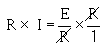Result of step 2: R x I = E
To get R alone (on one side of the equation) divide both sides by I: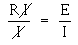The basic formula, transposed for R, is:Refer to figure 3-3 where E equals 10 volts and I equals 1 ampere. Solve for R, using the equation just explained.

Given:

E = 10 volts
I = 1 ampere

Solution:Figure 3-3. - Determining resistance in a basic circuit.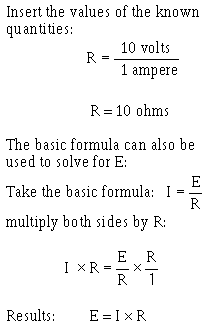This equation can be used to find the voltage for the circuit shown in figure 3-4.Figure 3-4. - Determining voltage in a basic circuit.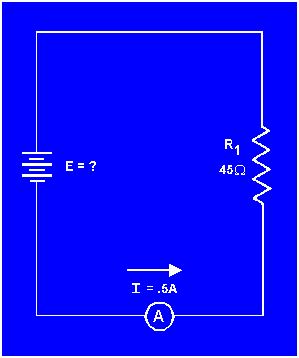The Ohm's law equation and its various forms may be obtained readily with the aid of figure 3-5. The circle containing E, I, and R is divided into two parts, with E above the line and with I and R below the line. To determine the unknown quantity, first cover that quantity with a finger. The position of the uncovered letters in the circle will indicate the mathematical operation to be performed. For example, to find I, cover I with a finger. The uncovered letters indicate that E is to be divided by R, orTo find the formula for E, cover E with your finger. The result indicates that I is to be multiplied by R, or E = IR. To find the formula for R, cover R. The result indicates that E is to be divided by I, orFigure 3-5. - Ohm's law in diagram form.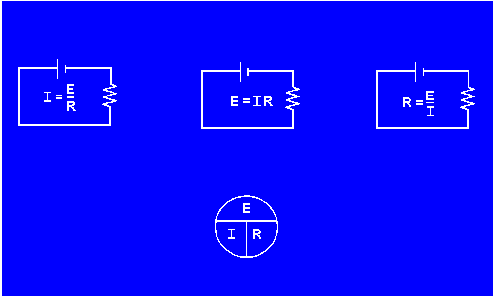You are cautioned not to rely wholly on the use of this diagram when you transpose the Ohm's law formulas. The diagram should be used to supplement your knowledge of the algebraic method. Algebra is a basic tool in the solution of electrical problems.

According to Ohm's law, what happens to circuit current
if the applied voltage (a) increases, (b) decreases?

According to Ohm's law, what happens to circuit current
if circuit resistance (a) increases, (b) decreases?

What is the equation used to find circuit resistance
if voltage and current values are known?Integrated Publishing, Inc. - A (SDVOSB) Service Disabled Veteran Owned Small Business